# Probability: Types of Events

Events can be Independent, Mutually Exclusive or Conditional !

Life is full of random events!
You need to get a "feel" for them to be a smart and successful person.
The toss of a coin, throw of a dice and lottery draws are all examples of random events.

## Events

When we say "Event" we mean one (or more) outcomes.
Example Events:
• Getting a Tail when tossing a coin is an event
• Rolling a "5" is an event.
An event can include several outcomes:
• Choosing a "King" from a deck of cards (any of the 4 Kings) is also an event
• Rolling an "even number" (2, 4 or 6) is an event

## Independent Events

Events can be "Independent", meaning each event is not affected by any other events.
This is an important idea! A coin does not "know" that it came up heads before ... each toss of a coin is a perfect isolated thing.
Example: You toss a coin three times and it comes up "Heads" each time ... what is the chance that the next toss will also be a "Head"?
The chance is simply 1/2, or 50%, just like ANY OTHER toss of the coin.
What it did in the past will not affect the current toss!
Some people think "it is overdue for a Tail", but really truly the next toss of the coin is totally independent of any previous tosses.
Saying "a Tail is due", or "just one more go, my luck is due" is called The Gambler's Fallacy

## Dependent Events

But some events can be "dependent" ... which means they can be affected by previous events ...

### Example: Drawing 2 Cards from a Deck

After taking one card from the deck there are less cards available, so the probabilities change!

Let's say you are interested in the chances of getting a King.
For the 1st card the chance of drawing a King is 4 out of 52
But for the 2nd card:
• If the 1st card was a King, then the 2nd card is less likely to be a King, as only 3 of the 51 cards left are Kings.
• If the 1st card was not a King, then the 2nd card is slightly more likely to be a King, as 4 of the 51 cards left are King.
This is because you are removing cards from the deck.
Replacement: When you put each card back after drawing it the chances don't change, as the events are independent.
Without Replacement: The chances will change, and the events are dependent.

## Tree Diagrams

When you have Dependent Events it helps to make a "Tree Diagram"

### Example: Soccer Game

You are off to soccer, and love being the Goalkeeper, but that depends who is the Coach today:
• with Coach Sam your probability of being Goalkeeper is 0.5
• with Coach Alex your probability of being Goalkeeper is 0.3
Sam is Coach more often ... about 6 of every 10 games (a probability of 0.6).

Let's build the Tree Diagram!
Start with the Coaches. We know 0.6 for Sam, so it must be 0.4 for Alex (the probabilities must add to 1):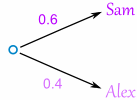Then fill out the branches for Sam (0.5 Yes and 0.5 No), and then for Alex (0.3 Yes and 0.7 No):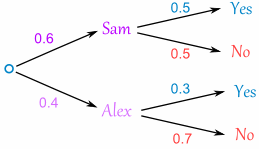## Mutually Exclusive

Mutually Exclusive means you can't get both events at the same time.
It is either one or the other, but not both
Examples:
• Turning left or right are Mutually Exclusive (you can't do both at the same time)
• Heads and Tails are Mutually Exclusive
• Kings and Aces are Mutually Exclusive
What isn't Mutually Exclusive
• Kings and Hearts are not Mutually Exclusive, because you can have a King of Hearts!
Like here: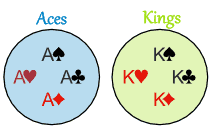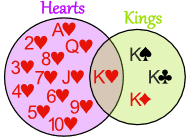Aces and Kings areMutually Exclusive Hearts and Kings are not Mutually Exclusive

# Mutually Exclusive Events

Mutually Exclusive: can't happen at the same time.
Examples:
• Turning left and turning right are Mutually Exclusive (you can't do both at the same time)
• Tossing a coin: Heads and Tails are Mutually Exclusive
• Cards: Kings and Aces are Mutually Exclusive
What is not Mutually Exclusive:
• Turning left and scratching your head can happen at the same time
• Kings and Hearts, because you can have a King of Hearts!
Like here:Aces and Kings areMutually Exclusive(can't be both) Hearts and Kings are not Mutually Exclusive(can be both)

## Probability

Let's look at the probabilities of Mutually Exclusive events. But first, a definition:
 Probability of an event happening = Number of ways it can happen Total number of outcomes
Example: there are 4 Kings in a deck of 52 cards. What is the probability of picking a King?
Number of ways it can happen: 4 (there are 4 Kings)
Total number of outcomes: 52 (there are 52 cards in total)
 So the probability = 4 = 1 52 13

## Mutually Exclusive

When two events (call them "A" and "B") are Mutually Exclusive it is impossible for them to happen together:
P(A and B) = 0
"The probability of A and B together equals 0 (impossible)"

But the probability of A or B is the sum of the individual probabilities:
P(A or B) = P(A) + P(B)
"The probability of A or B equals the probability of A plus the probability of B"

### Example: A Deck of Cards

In a Deck of 52 Cards:
• the probability of a King is 1/13, so P(King)=1/13
• the probability of an Ace is also 1/13, so P(Ace)=1/13

When we combine those two Events:
• The probability of a card being a King and an Ace is 0 (Impossible)
• The probability of a card being a King or an Ace is (1/13) + (1/13) = 2/13
Which is written like this:
P(King and Ace) = 0
P(King or Ace) = (1/13) + (1/13) = 2/13

## Special Notation

Instead of "and" you will often see the symbol  (which is the "Intersection" symbol used in Venn Diagrams)
Instead of "or" you will often see the symbol  (the "Union" symbol)### Example: Scoring Goals

If the probability of:
• scoring no goals (Event "A") is 20%
• scoring exactly 1 goal (Event "B") is 15%
Then:
• The probability of scoring no goals and 1 goal is 0 (Impossible)
• The probability of scoring no goals or 1 goal is 20% + 15% = 35%

Which is written:
P(A  B) = 0
P(A  B) = 20% + 15% = 35%

## Remembering

"Or has more ... than And"
is like a cup which holds more than

## Not Mutually Exclusive

Now let's see what happens when events are not Mutually Exclusive.

### Example: Hearts and KingsHearts and Kings together is only the King of Hearts: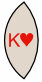But Hearts or Kings is:
• all the Hearts (13 of them)
• all the Kings (4 of them)
But that counts the King of Hearts twice!
So we correct our answer, by subtracting the extra "and" part: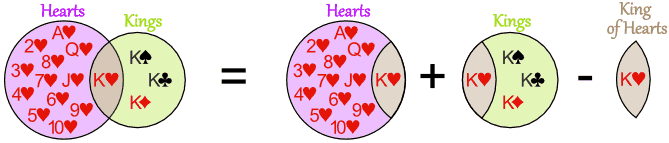16 Cards = 13 Hearts + 4 Kings - the 1 extra King of Hearts
Count them to make sure this works!
As a formula this is:
P(A or B) = P(A) + P(B) - P(A and B)
"The probability of A or B equals the probability of A plus the probability of B
minus the probability of A and B"
Here is the same formula, but using  and :
P(A ∪ B) = P(A) + P(B) - P(A ∩ B)

## A Final Example

16 people study French, 21 study Spanish and there are 30 altogether. Work out the probabilities!
This is definitely a case of not Mutually Exclusive (you can study French AND Spanish).
Let's say b is how many study both languages:
• people studying French Only must be 16-b
• people studying Spanish Only must be 21-b
And we get: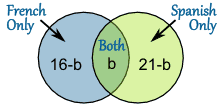And we know there are 30 people, so:
(16-b) + b + (21-b) = 30
37 - b = 30
b = 7
And we can put in the correct numbers: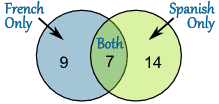So we know all this now:
• P(French) = 16/30
• P(Spanish) = 21/30
• P(French Only) = 9/30
• P(Spanish Only) = 14/30
• P(French or Spanish) = 30/30 = 1
• P(French and Spanish) = 7/30
Lastly, let's check with our formula:
P(A or B) = P(A) + P(B) - P(A and B)
Put the values in:
30/30 = 16/30 + 21/30 – 7/30
Yes, it works!

## Summary:

### Mutually Exclusive

• and B together is impossible: P(A and B) = 0
• or B is the sum of A and B: P(A or B) = P(A) + P(B)

### Not Mutually Exclusive

• or B is the sum of A and B minus A and B: P(A or B) = P(A) + P(B) - P(A and B)
• # Probability: Independent Events

Life is full of random events!
You need to get a "feel" for them to be a smart and successful person.
The toss of a coin, throwing dice and lottery draws are all examples of random events.
Sometimes an event can affect the next event.
Example: taking colored marbles from a bag: as you take each marble there are less marbles left in the bag, so the probabilities change.
We call those Dependent Events, because what happens depends on what happened before .
But otherwise they are Independent Events ...

## Independent Events

 Independent Events are not affected by previous events. This is an important idea! A coin does not "know" it came up heads before ... .... each toss of a coin is a perfect isolated thing.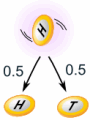### Example: You toss a coin and it comes up "Heads" three times ... what is the chance that the next toss will also be a "Head"?

The chance is simply ½ (or 0.5) just like ANY toss of the coin.
What it did in the past will not affect the current toss!

Some people think "it is overdue for a Tail", but really truly the next toss of the coin is totally independent of any previous tosses.
Saying "a Tail is due", or "just one more go, my luck is due" is called The Gambler's Fallacy
Of course your luck may change, because each toss of the coin has an equal chance.

## Probability of Independent Events

"Probability" (or "Chance") is how likely something is to happen.
So how do we calculate probability?
 Probability of an event happening = Number of ways it can happen Total number of outcomes### Example: what is the probability of getting a "Head" when tossing a coin?

Number of ways it can happen: 1 (Head)
Total number of outcomes: 2 (Head and Tail)
 So the probability = 1 = 0.5 2

### Example: what is the probability of getting a "5" or "6" when rolling a die?

Number of ways it can happen: 2 ("5" and "6")
Total number of outcomes: 6 ("1", "2", "3", "4", "5" and "6")
 So the probability = 2 = 1 = 0.333... 6 3

## Ways of Showing Probability

Probability goes from 0 (imposssible) to 1 (certain):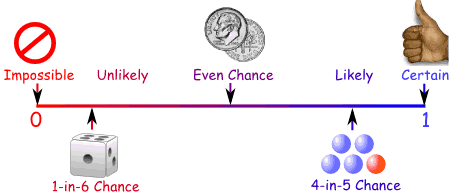It is often shown as a decimal or fraction.
Example: the probability of getting a "Head" when tossing a coin:
• As a decimal: 0.5
• As a fraction: 1/2
• As a percentage: 50%
• Or sometimes like this: 1-in-2

## Two or More Events

You can calculate the chances of two or more independent events by multiplying the chances.

### Example: Probability of 3 Heads in a Row

For each toss of a coin a "Head" has a probability of 0.5: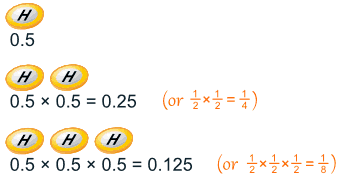And so the chance of getting 3 Heads in a row is 0.125
So each toss of a coin has a ½ chance of being Heads, but lots of Heads in a row is unlikely.

### Example: Why is it unlikely to get, say, 7 heads in a row, when each toss of a coin has a ½ chance of being Heads?

Because you are asking two different questions:
Question 1: What is the probability of 7 heads in a row?
Answer: ½×½×½×½×½×½×½ = 0.0078125 (less than 1%).
Question 2: Given that you have just got 6 heads in a row, what is the probability that the next toss is also a head?
Answer: ½, as the previous tosses don't affect the next toss.
You can have a play with the Quincunx to see how lots of independent effects can still have a pattern.

## Notation

We use "P" to mean "Probability Of",
So, for Independent Events:
P(A and B) = P(A) × P(B)
Probability of A and B equals the probability of A times the probability of B

### Example: you are going to a concert, and your friend says it is some time on the weekend between 4 and 12, but won't say more.

What are the chances it is on Sunday between 10 and 12?

Day: there are two days on the weekend, so P(Sunday) = 0.5
Time: between 4 and 12 is 8 hours, but you want between 10 and 12 which is only 2 hours:
P(Your Time) = 2/8 = 0.25
And:
P(Sunday and Your Time) = P(Sunday) × P(Your Time) = 0.5 × 0.25 = 0.125
Or a 12.5% chance

## Another Example

Imagine there are two groups:
• A member of each group gets randomly chosen for the winners circle,
• then one of those gets randomly chosen to get the big money prize: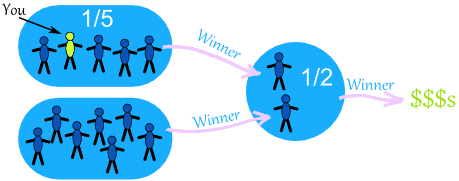What is your chance of winnning the big prize?
• there is a 1/5 chance of going to the winners circle
• and a 1/2 chance of winning the big prize
So you have a 1/5 chance followed by a 1/2 chance ... which makes a 1/10 chance overall:
 1 × 1 = 1 = 1 5 2 5 × 2 10
Or you can calculate using decimals (1/5 is 0.2, and 1/2 is 0.5):
0.2 x 0.5 = 0.1
So your chance of winning the big money is 0.1 (which is the same as 1/10).

## Coincidence!

Many "Coincidences" are, in fact, likely.

### Example: you are in a room with 30 people, and find that Zach and Anna celebrate their birthday on the same day.

Would you say "wow, how strange", or "that seems reasonable, with so many people here".
In fact there is a 70% chance that would happen ... so it is likely.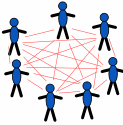Why is the chance so high? Because you are comparing everyone to everyone else (not just one to many). And with 30 people that is 435 comparisons
(Read Shared Birthdays to find out more.)

### Example: Snap!

Did you ever say something the same as someone else, at the same time too?
Wow, how amazing!
But you were probably sharing an experience (movie, journey, whatever) and so your thoughts would be similar.
And there are only so many ways of saying something ...
... so it is like the card game "Snap!" ...
... if you speak enough words together, they will eventually match up.
So, maybe not so amazing, just simple chance at work.
Can you think of other cases where a "coincidence" was simply a likely thing?

## Conclusion

• Probability is: (Number of ways it can happen) / (Total number of outcomes)
• Dependent Events (such as removing marbles from a bag) are affected by previous events
• Independent events (such as a coin toss) are not affected by previous events
• You can calculate the probability of 2 or more Independent events by multiplying
• Not all coincidences are really unlikely (when you think about them).

# Conditional Probability

How to handle Dependent Events
Life is full of random events! You need to get a "feel" for them to be a smart and successful person.

## Independent Events

Events can be "Independent", meaning each event is not affected by any other events.

### Example: Tossing a coin.

Each toss of a coin is a perfect isolated thing.
What it did in the past will not affect the current toss.
The chance is simply 1-in-2, or 50%, just like ANY toss of the coin.
So each toss is an Independent Event.

## Dependent Events

But events can also be "dependent" ... which means they can be affected by previous events ...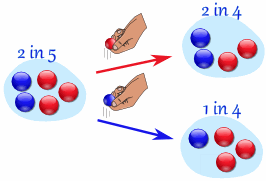### Example: Marbles in a Bag

2 blue and 3 red marbles are in a bag.
What are the chances of getting a blue marble?
The chance is 2 in 5
But after taking one out you change the chances!
So the next time:
• if you got a red marble before, then the chance of a blue marble next is 2 in 4
• if you got a blue marble before, then the chance of a blue marble next is 1 in 4
See how the chances change each time? Each event depends on what happened in the previous event, and is called dependent.
That is the kind of thing we will be looking at here.

"Replacement"
Note: if you had replaced the marbles in the bag each time, then the chances would not have changed and the events would be independent:
• With Replacement: the events are Independent (the chances don't change)
• Without Replacement: the events are Dependent (the chances change)

## Tree Diagram

A Tree Diagram: is a wonderful way to picture what is going on, so let's build one for our marbles example.
There is a 2/5 chance of pulling out a Blue marble, and a 3/5 chance for Red: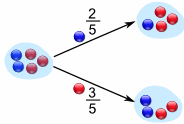We can even go one step further and see what happens when we select a second marble: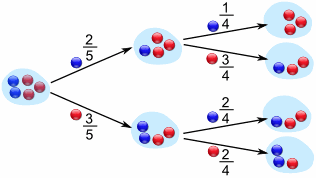If a blue marble was selected first there is now a 1/4 chance of getting a blue marble and a 3/4 chance of getting a red marble.
If a red marble was selected first there is now a 2/4 chance of getting a blue marble and a 2/4 chance of getting a red marble.
Now we can answer questions like "What are the chances of drawing 2 blue marbles?"
Answer: it is a 2/5 chance followed by a 1/4 chance: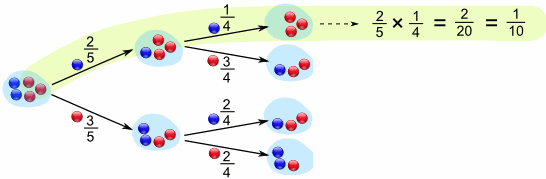Did you see how we multiplied the chances? And got 1/10 as a result.
The chances of drawing 2 blue marbles is 1/10

## Notation

We love notation in mathematics! It means we can then use the power of algebra to play around with the ideas. So here is the notation for probability:
P(A) means "Probability Of Event A"
In our marbles example Event A is "get a Blue Marble first" with a probability of 2/5:
P(A) = 2/5
And Event B is "get a Blue Marble second" ... but for that we have 2 choices:
• If we got a Blue Marble first the chance is now 1/4
• If we got a Red Marble first the chance is now 2/4
So we have to say which one we want, and use the symbol "|" to mean "given":
P(B|A) means "Event B given Event A"
In other words, event A has already happened, now what is the chance of event B?
P(B|A) is also called the "Conditional Probability" of B given A.
And in our case:
P(B|A) = 1/4
So the probability of getting 2 blue marbles is: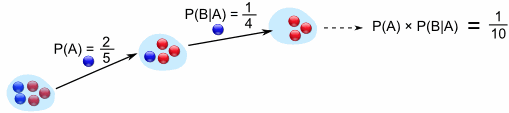And we write it as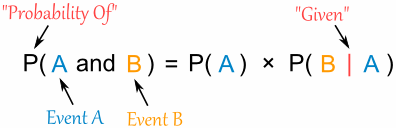"Probability of event A and event B equals
the probability of event A times the probability of event B given event A"

Let's do the next example using only notation:

### Example: Drawing 2 Kings from a Deck

Event A is drawing a King first, and Event B is drawing a King second.
For the first card the chance of drawing a King is 4 out of 52
P(A) = 4/52
But after removing a King from the deck the probability of the 2nd card drawn is less likely to be a King (only 3 of the 51 cards left are Kings):
P(B|A) = 3/51
And so:
P(A and B) = P(A) x P(B|A) = (4/52) x (3/51) = 12/2652 = 1/221
So the chance of getting 2 Kings is 1 in 221, or about 0.5%

## Finding Hidden Data

Using Algebra we can also "change the subject" of the formula, like this:
 Start with: P(A and B) = P(A) x P(B|A) Swap sides: P(A) x P(B|A) = P(A and B) Divide by P(A): P(B|A) = P(A and B) / P(A)
And we have another useful formula:"The probability of event B given event A equals
the probability of event A and event B divided by the probability of event A

### Example: Ice Cream

70% of your friends like Chocolate, and 35% like Chocolate AND like Strawberry.
What percent of those who like Chocolate also like Strawberry?
P(Strawberry|Chocolate) = P(Chocolate and Strawberry) / P(Chocolate)
0.35 / 0.7 = 50%
50% of your friends who like Chocolate also like Strawberry## Big Example: Soccer Game

You are off to soccer, and want to be the Goalkeeper, but that depends who is the Coach today:
• with Coach Sam the probability of being Goalkeeper is 0.5
• with Coach Alex the probability of being Goalkeeper is 0.3
Sam is Coach more often ... about 6 out of every 10 games (a probability of 0.6).
So, what is the probability you will be a Goalkeeper today?

Let's build a tree diagram. First we show the two possible coaches: Sam or Alex:The probability of getting Sam is 0.6, so the probability of Alex must be 0.4 (together the probability is 1)
Now, if you get Sam, there is 0.5 probability of being Goalie (and 0.5 of not being Goalie):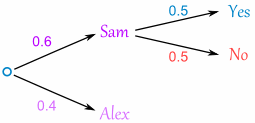If you get Alex, there is 0.3 probability of being Goalie (and 0.7 not):The tree diagram is complete, now let's calculate the overall probabilities. Remember that:
P(A and B) = P(A) x P(B|A)
Here is how to do it for the "Sam, Yes" branch: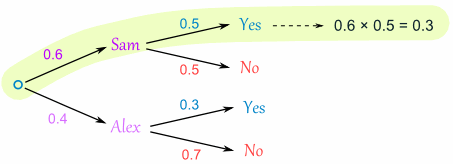(When we take the 0.6 chance of Sam being coach and include the 0.5 chance that Sam will let you be Goalkeeper we end up with an 0.3 chance.)
But we are not done yet! We haven't included Alex as Coach: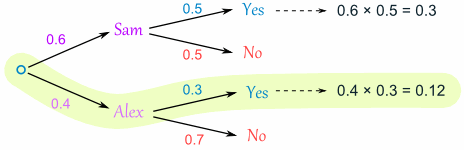An 0.4 chance of Alex as Coach, followed by an 0.3 chance gives 0.12
And the two "Yes" branches of the tree together make:
0.3 + 0.12 = 0.42 probability of being a Goalkeeper today
(That is a 42% chance)

### Check

One final step: complete the calculations and make sure they add to 1: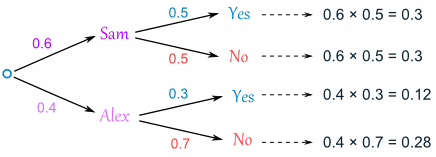0.3 + 0.3 + 0.12 + 0.28 = 1
Yes, they add to 1, so that looks right.

## Friends and Random Numbers

Here is another quite different example of Conditional Probability.
4 friends (Alex, Blake, Chris and Dusty) each choose a random number between 1 and 5. What is the chance that any of them chose the same number?
Let's add our friends one at a time ...

### First, what is the chance that Alex and Blake have the same number?

Blake compares his number to Alex's number. There is a 1 in 5 chance of a match.
As a tree diagram: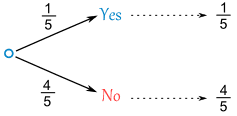Note: "Yes" and "No" together  makes 1
(1/5 + 4/5 = 5/5 = 1)

### Now, let's include Chris ...

But there are now two cases to consider:
• If Alex and Billy did match, then Chris has only one number to compare to.
• But if Alex and Billy did not match then Chris has two numbers to compare to.
And we get this: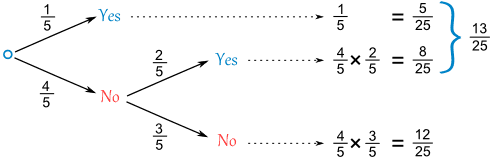For the top line (Alex and Billy did match) we already have a match (a chance of 1/5).
But for the "Alex and Billy did not match" there is now a 2/5 chance of Chris matching (because Chris gets to match his number against both Alex and Billy).
And we can work out the combined chance by multiplying the chances it took to get there:
Following the "No, Yes" path ... there is a 4/5 chance of No, followed by a 2/5 chance of Yes:
(4/5) × (2/5) = 8/25
Following the "No, No" path ... there is a 4/5 chance of No, followed by a 3/5 chance of No:
(4/5) × (3/5) = 12/25
Also notice that when you add all chances together you still get 1 (a good check that we haven't made a mistake):
(5/25) + (8/25) + (12/25) = 25/25 = 1

### Now what happens when we include Dusty?

It is the same idea, just more of it: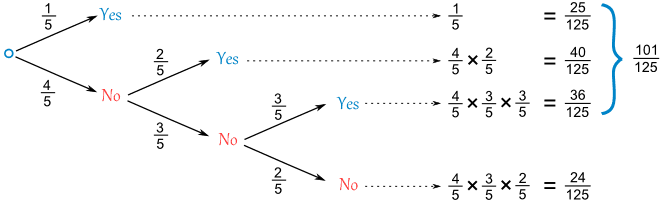OK, that is all 4 friends, and the "Yes" chances together make 101/125:

But notice something interesting ... if we had followed the "No" path we could have skipped all the other calculations and made our life easier: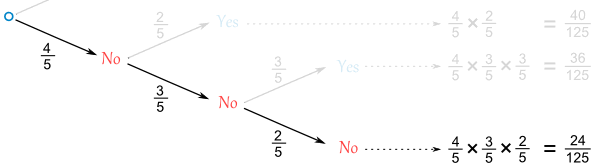The chances of not matching are:
(4/5) × (3/5) × (2/5) = 24/125
So the chances of matching are:
1 - (24/125) = 101/125
(And we didn't really need a tree diagram for that!)

# Probability Tree Diagrams

Calculating probabilities can be hard, sometimes you add them, sometimes you multiply them, and often it is hard to figure out what to do ... tree diagrams to the rescue!

Here is a tree diagram for the toss of a coin:There are two "branches" (Heads and Tails) The probability of each branch is written on the branch The outcome is written at the end of the branch
We can extend the tree diagram to two tosses of a coin: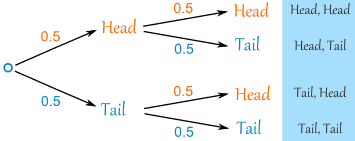How do you calculate the overall probabilities?
• You multiply probabilities along the branches
• You add probabilities down columns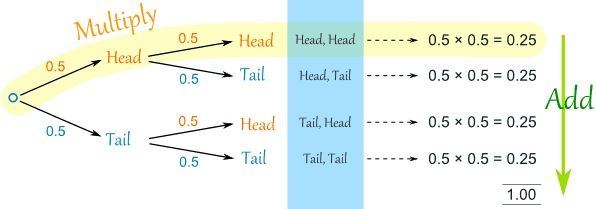Now we can see such things as:
• The probability of "Head, Head" is 0.5×0.5 = 0.25
• All probabilities add to 1.0 (which is always a good check)
• The probability of getting at least one Head from two tosses is 0.25+0.25+0.25 = 0.75
• ... and more

That was a simple example using independent events (each toss of a coin is independent of the previous toss), but tree diagrams are really wonderful for figuring out dependent events (where an event depends on what happens in the previous event) like this example:## Example: Soccer Game

You are off to soccer, and love being the Goalkeeper, but that depends who is the Coach today:
• with Coach Sam the probability of being Goalkeeper is 0.5
• with Coach Alex the probability of being Goalkeeper is 0.3
Sam is Coach more often ... about 6 out of every 10 games (a probability of 0.6).
So, what is the probability you will be a Goalkeeper today?

Let's build the tree diagram. First we show the two possible coaches: Sam or Alex:The probability of getting Sam is 0.6, so the probability of Alex must be 0.4 (together the probability is 1)
Now, if you get Sam, there is 0.5 probability of being Goalie (and 0.5 of not being Goalie):If you get Alex, there is 0.3 probability of being Goalie (and 0.7 not):The tree diagram is complete, now let's calculate the overall probabilities. This is done by multiplying each probability along the "branches" of the tree.
Here is how to do it for the "Sam, Yes" branch:(When we take the 0.6 chance of Sam being coach and include the 0.5 chance that Sam will let you be Goalkeeper we end up with an 0.3 chance.)
But we are not done yet! We haven't included Alex as Coach:An 0.4 chance of Alex as Coach, followed by an 0.3 chance gives 0.12.
Now we add the column:
0.3 + 0.12 = 0.42 probability of being a Goalkeeper today
(That is a 42% chance)

### Check

One final step: complete the calculations and make sure they add to 1:0.3 + 0.3 + 0.12 + 0.28 = 1
Yes, it all adds up.

## Conclusion

So there you go, when in doubt draw a tree diagram, multiply along the branches and add the columns. Make sure all probabilities add to 1 and you are good to go.